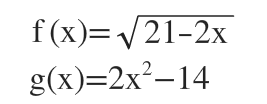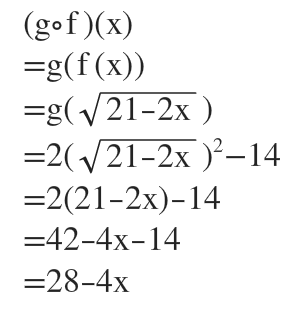# f(x)=√21−2x  g(x)=2x^2−14.(g∘f)(x)the question is what is the domain?

Question
2 views

f(x)=√21−2x  g(x)=2x^2−14.

(g∘f)(x)

the question is what is the domain?

check_circle

Step 1We have to plug f(x)=sqrt(21-2x) inside g(x) and simplify....

### Want to see the full answer?

See Solution

#### Want to see this answer and more?

Solutions are written by subject experts who are available 24/7. Questions are typically answered within 1 hour.*

See Solution
*Response times may vary by subject and question.
Tagged in